﻿ Do We Really Need the Concentration and Volume of a Solution of a Weak Acid to Calculate the Percentage Ionization or Degree of Ionization?Publications are Open
Access in this journal
Article Versions
Export Article
• Normal Style
• MLA Style
• APA Style
• Chicago Style
Research Article
Open Access Peer-reviewed

### Do We Really Need the Concentration and Volume of a Solution of a Weak Acid to Calculate the Percentage Ionization or Degree of Ionization?

R. Sanjeev, P. Sreedhar, V. Jagannadham
World Journal of Chemical Education. 2021, 9(2), 64-67. DOI: 10.12691/wjce-9-2-5
Received July 02, 2021; Revised August 05, 2021; Accepted August 16, 2021

### Abstract

In the present work a simple and lucid method of calculation of percentage ionization or degree of ionization is explained.

### 1. Introduction

Percentage ionization or degree of ionization of weak acids play a vital role in the drug absorption in to the blood stream 1. Therefore it is necessary for 12th grade high school students and college freshmen students of biology majors to understand the importance of percentage ionization or degree of ionization of a weak acid. In the present article the use of KaleidaGraph software is presented and also we reported similar exercise earlier 2.

### 2. Methods

All calculations and curve fittings were done by Kaleida Graph software version 4.1 supplied by Synergy Software INC., Reading, PA, USA.

### 3. Discussion

By definition the percentage ionization of any species like weak acid (HA(aq) ⇌ H+(aq)+ A-(aq)) could be written as: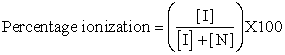(1)

Here [I] is concentration of ionized species i.e. of [H+] or [A-] and [N] is that of non-ionized species i.e. of [HA] at equilibrium.

Hence to calculate the percentage ionization of weak acid one needs the [I] and [N] at equilibrium. No doubt the quantity {[I] + [N]} is the total initial concentration of the weak acid. Therefore the student in a class has to weigh the required quantity of the acid if it is a solid or he has to take required volume of the acid if it is a liquid. [I] could be obtained from the measured pH of the solution since at equilibrium [H+] = [A-]. Hence, the student must do an extra work of weighing in the laboratory in addition to measuring the pH of the solution. Here the pKa of the weak acid has to do nothing.

However one needs two quantities at a given instance to calculate the percentage or degree of ionization of the weak acid at equilibrium:

Equation 1 gives the percentage ionization. The degree of ionization (α) is calculated as follows:

Let the following equilibrium reaction depicts the dissociation of a weak acid (HA) in water.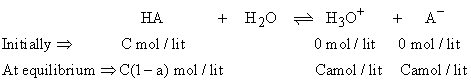(2)

As shown above, let “C” be the initial concentration in mol/lit of the acid, HA and zero mol/lit of the H3O+ and A- each. And let “α” be the degree of ionization. Therefore at equilibrium the concentrations of HA, H3O+ and A- are C(1-α), Cα and Cα respectively.

Therefore K the equilibrium constant is given by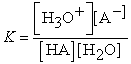(3)

or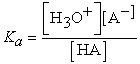(4)

Where Ka is the dissociation constant of the weak acid.

Therefore in terms of equilibrium concentrations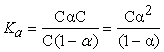(5)

Therefore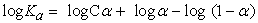(6)

or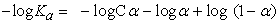(7)

Therefore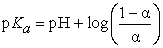(8)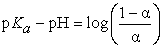(9)

or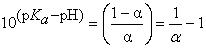(10)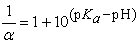(11)

or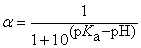(12)

Therefore from eqn. 1 it is clear that one needs the initial concentration of the acid to calculate the percentage ionization. And from eqn. 12 it is clear that one needs the pH and the pKa of the acid. Eqn. 12 doesn’t involve any laboratory weighing of the substance.

As an example in the classroom work, if the degree of ionization (α) is given at different pH, students can draw graph α versus pH using KaleidaGraph software. The table and graph are shown in the following taking acetic acid as an example with the simulated data.

• Figure 1. Plot of degree of ionization (α) versus pH

One can buy KaleidaGraph software version 4.5 from Synergy Software Company, Reading PA, USA. It is very user-friendly and hands-on tool for 12th grade high school students and college freshmen students. The chronological order of using the program is given below:

Step 1: Open the KaleidaGraph program. An empty window will be popped up like the one shown in Figure 2

Step 2: Then one can fill the data under column A for “X” coordinate and in column B for “Y” coordinate. By clicking “A” and “B” one can name the coordinates as pH and α. Then the Figure 3 appears

Step 3: In Figure 3, click “gallery”, then click “linear” and then click “scatter”. A window like the one shown in Figure 4 will appear.

Step 4: Then click “X” for pH and “Y” for alpha. A window as shown in Figure 5 will appear

Step 5: Then click “new plot” in Figure 5. A graph as shown in Figure 6 will appear.

Step 6: In Figure 6 click “curve fit”, then click “general” and “fit1”. Then pop up window shown in Figure 7 will appear.

Step 7: Then click “Define”. A pop up window as shown in Figure 8 will appear.

Step 8: In Figure 8 under the General curve fit one can type the required equation as shown in Figure 9. Here m1 is an arbitrary constant stands for “pKa” and m0 stands for “X” variable.

Step 9: Then click OK in Figure 9.

Step 10: The final graph as shown in Figure 10 will appear. After editing it looks like as shown in Figure 1 at the top.

### References

  Robert G. Lamb, www2.courses.vcu.edu/ptxed/.../Lamb%20Drug%20Absorption.PDF. In article  The KaleidaGraph and the Kontron: “Hands On” Tools in a Physical Chemistry Laboratory for Determination of pKa of Weak Acids and Weak Bases, V. Jagannadham, and R. Sanjeev, Advances in Analytical Chemistry, Published by Scientific and Academic Publishing, USA, (2013) Vol. 3, page 54-56. In articleThis work is licensed under a Creative Commons Attribution 4.0 International License. To view a copy of this license, visit http://creativecommons.org/licenses/by/4.0/